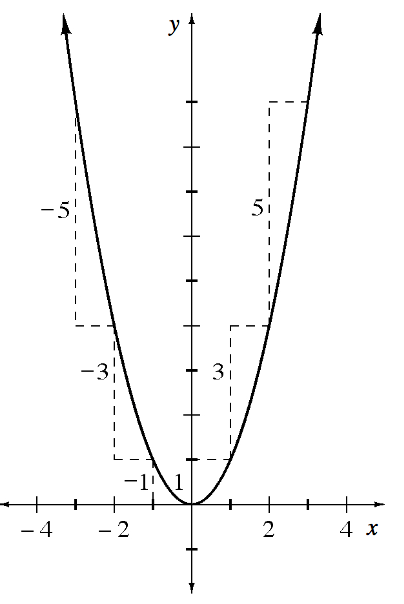### Home > APCALC > Chapter 2 > Lesson 2.2.2 > Problem2-68

2-68.

If you plot the finite differences of a parabola, the result will be what type of function?

Review problem 1-96.

Recall that $\Delta y$ represents the finite difference. What pattern do you see among the finite differences of the parabola $y = x^2$? What would the graph of those finite difference look like? Is this true for all parabolas?$\boldsymbol x$ $-3$ $-2$ $-1$ $0$ $1$ $2$ $3$ $\boldsymbol{f(x)}$ $9$ $4$ $1$ $0$ $1$ $4$ $9$ $\boldsymbol{\Delta{y}}$ $\underset{\LARGE{\smile}}{-5}$ The difference between 9 and 4 is minus 5          $\underset{\LARGE{\smile}}{-3}$  The difference between 4 and 1 is minus 3         $\underset{\LARGE{\smile}}{-1}$ The difference between 1 and 0 is minus 1.         $\underset{\LARGE{\smile}}{+1}$The difference between 0 and 1 is positive 1.           $\underset{\LARGE{\smile}}{+3}$ The difference between 1 and 4 is positive 3.           $\underset{\LARGE{\smile}}{+5}$ The difference between 4 and 9 is positive 5.